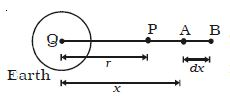Physics

# Explain Gravitational Potential Energy

Consider a body of mass m placed at P at a distance r from the centre of the Earth. Let the mass of the Earth be M.When the mass m is at A at a distance x from Q, the gravitational force of attraction on it due to mass M is given by F = GMm/x2

The work done in moving the mass m through a small distance dx from A to B along the line joining the two centres of masses m and M is duv = -F.dx

duv = – (GMm/x2) . dx

The gravitational potential energy of a mass m at a distance r from another mass M is defined as the amount of work done in moving the mass m from a distance r to infinity.

The total work done in moving the mass m from a distance r to inutility is:

∫ dw = – r GM/x2  dx

W = – GMm r 1/x2 dx

So, U = – GMm/r

Gravitational potential energy is zero at infinity and decreases as the distance decreases. This is due to the fact that the gravitational force exerted on the body by the Earth is attractive. Hence the gravitational potential energy U is negative.